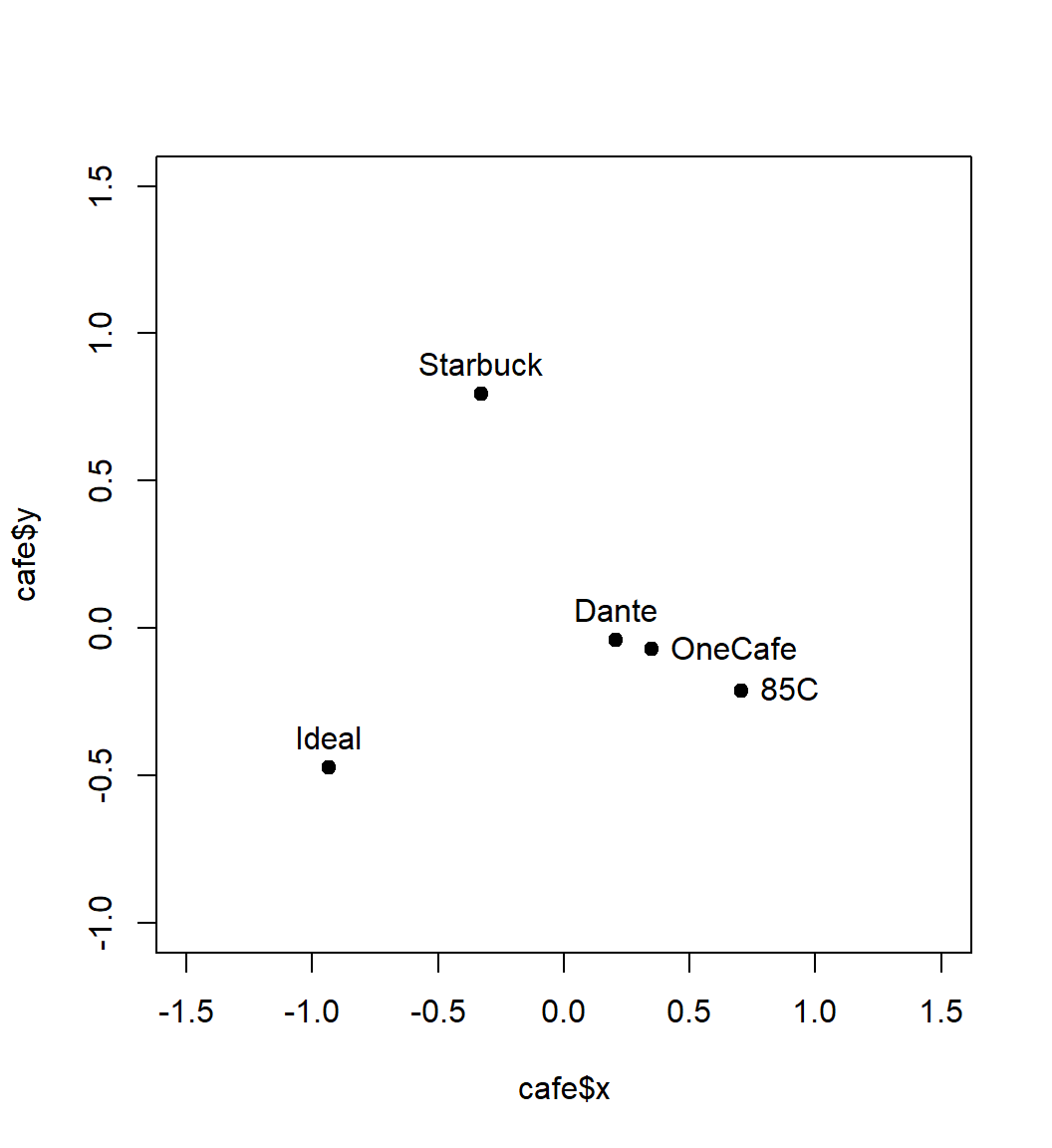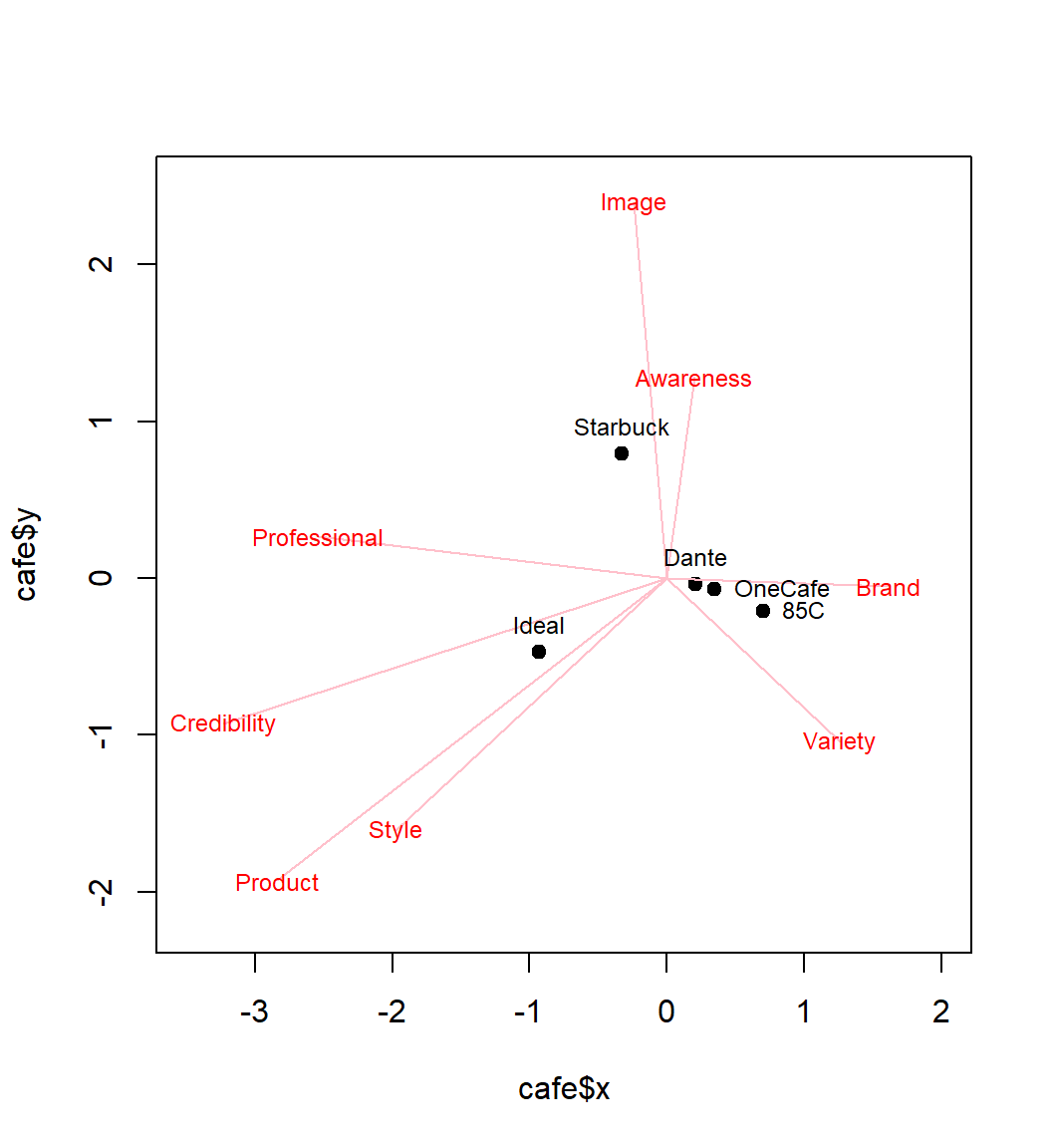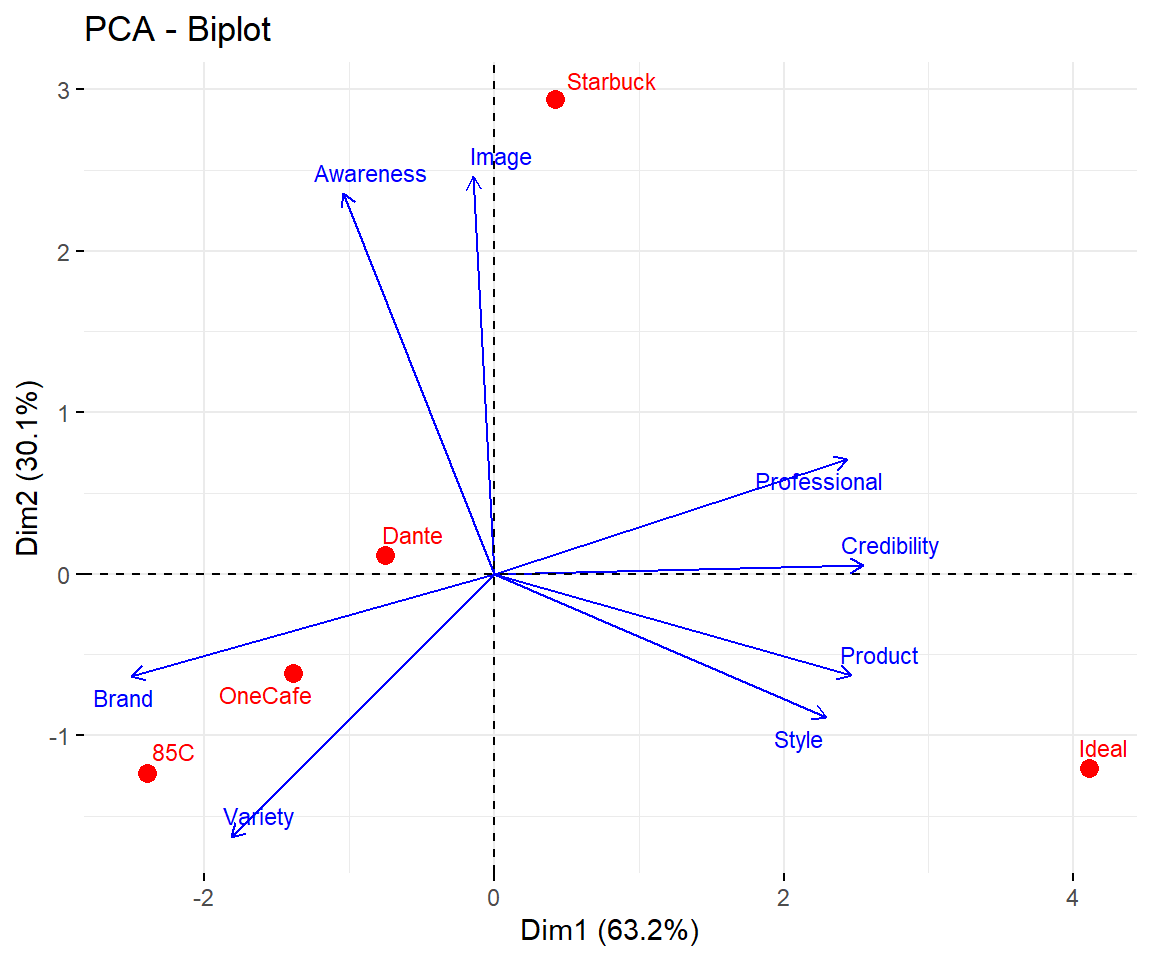💡 KEY LEARNINGS：
■ MDS - Multi-Dimension Scaling
§ a different method for dimension reduction
§ try to maintain the distance in low dimension space
■ Perception Map - a marketing tool that visualize …
§ The similarity among the brands
§ The correlation among the brand attributes
§ The relationship between the brands and the attributes

pacman::p_load(dplyr, FactoMineR, factoextra)
##### § Reading and Preapring Data
load("data/cafe.rdata")
row.names(cafe) = cafe[,1]
cafe = cafe[,-1]

names(cafe) = c(
"Variety","Style","Product","Image",
"Brand","Awareness","Professional","Credibility")
rownames(cafe) = c("Dante","Starbuck","85C","OneCafe","Ideal")
cafe
         Variety Style Product Image Brand Awareness Professional Credibility
Dante       3.10  2.55    2.85  2.98  2.28      2.66         2.42        2.22
Starbuck    2.35  2.68    2.84  3.21  2.07      3.27         2.62        2.22
85C         3.23  2.69    2.79  2.77  2.73      2.65         2.13        1.99
OneCafe     2.90  2.62    2.74  2.70  2.57      2.66         2.41        2.09
Ideal       2.56  3.17    3.19  2.71  1.72      2.23         2.81        2.50

We don’t have much data. Just 8 attributes and 5 brands. Yet it is still challenging to compare all of the 8 attributes on this 5 brand at once.

### 1. Multi-Dimensional Scaling (MDS)

• Calculate the distance matrix by dist()
• Call cmdscale() for MDS analysis.
d = dist(cafe, method = "euclidean")
fit <- cmdscale(d, eig = TRUE, k = 2)
• Then plot the brands on the space of reduced dimensions.
brands = rownames(cafe); percepts=colnames(cafe)
cafe$x = fit$points[, 1]
cafe$y = fit$points[, 2]
plot(cafe$x, cafe$y, pch = 19, xlim=c(-1.5,1.5), ylim=c(-1,1.5))
bpos = c(3, 3, 4, 4, 3)
text(cafe$x, cafe$y, pos=bpos, offset=0.5, labels = brands)Perceived difference among the brands are represented as the corresponding distances on the map.

### 2. Percpetual Map

Furthermore, we can also plot the unit vectors for the original dimension on the map.

plot(cafe$x, cafe$y, pch = 19, xlim=c(-3.5,2), ylim=c(-2.2,2.5))
for(i in 1:length(percepts)) {
m = lm(sprintf("%s ~ x + y",percepts[i]), data=cafe)
c = 1/m$coef; d = 1/m$coef
cd2 = c*c + d*d
px = (c * d * d)/cd2
py = (c * c * d)/cd2
arrows(0,0,px,py,col="pink",length=0)
text(px, py, col="red", cex=0.75, labels = percepts[i])
}
text(cafe$x, cafe$y, pos=bpos, offset=0.5, cex=0.75, labels = brands)### 3. PCA

The result of MDS is quite similar to that of PCA

PCA(cafe[1:8], graph=F) %>% fviz_pca_biplot(
repel=T, col.var="blue", col.ind="red",
labelsize=3, pointshape=16, pointsize=3)🗿 DISCUSSION 1:
■ What can you tell from the Perception Map?
■ Are these finding useful (for marketing)?

🗿 DISCUSSION 2:
■ Why do we do dimension reduction?
■ What is the common logic of dimension reduction algorithm?< br>   ■ What are the pro and con’s of dimension reduction?
■ What are the 3 important information we can learn from dimension reduction?